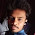## Tuesday, December 01, 2015

### Logarithms Basics - Natural and Common Logarithm, Converting Exponential form to Logarithmic Function, Characteristic and Mantissa, Logarithms Formulae

This video deals with the basics of Logarithms topic. The relation between three variables can be expressed through two forms of equations named Exponential Form of equation and Logarithmic Function. The theory has been written by me in the snapshot that I have uploaded below which has been made by me in order to make things easy for you. The same concepts written in the snapshot has also been explained by me in the video. Then after finishing with the concepts I have explained the application of these concepts in questions. The two types of Logarithms named Natural Logarithm and Common Logarithm has been detailed by me in the video. I have also told how to calculate the Characteristic and Mantissa of a Logarithmic Function. The mantissa of a logarithmic function will always be positive and can never be negative. Around 10 formulae of Logarithms have been detailed by me and these are those properties that are very important for solving almost all questions of Logarithms.

Question 6. If 3(4 - 3a) = 9(2a - 2), what is the value of a ?

Question 7. log(2m - 2) - log(11.66 - m) = 1 + log 3

Find the value of m here ?

1.sir these videos are not free

2.ur also commersialed

3.thanks a lot sir, for making available video lecture for each and every topic, this will surely help us all a lot.